# All About the Regression Learner App

These columns will look at a topic, explain some of the background, and answer a few questions that I’ve heard from the MATLAB® and Simulink® community.

This column is all about questions people have about the Regression Learner app. I’ll summarize what the app is, and then take a look at five questions:

#### 5. How can I make predictions with new data?

This column answers some questions about the Regression Learner app in MATLAB; it isn’t about regression in general.

The Regression Learner app has been available to engineers and scientists since 2017. Regression analysis helps people understand the relationship between variables and numeric responses and can be applied to tasks such as predicting energy consumption, financial performance, and manufacturing process parameters.

Fundamentally, the Regression Learner app enables you to build regression models interactively, without writing code, and measure the accuracy and performance of your models. You can quickly compare the performance of various regression models and features.

The app is especially useful for people getting started with machine learning, so I’m excited to answer some questions directly related to the app.

If you're unfamiliar with the Regression Learner app, here's a demo video to get you up to speed:
Watch video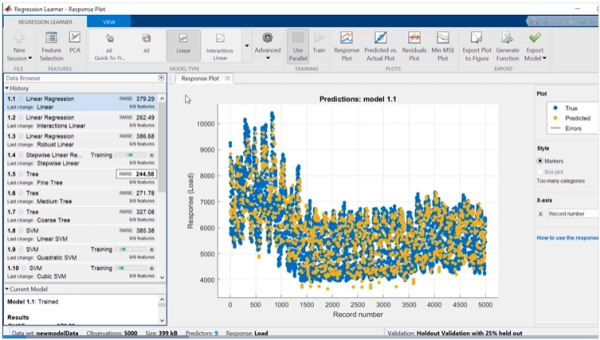QUESTION ONE

# Can I use the Regression Learner app with time-series data?

Absolutely. For example, you can use regression models to forecast electrical load or
predict damage costs from a storm
.

Like with other machine learning applications, it is important to preprocess and clean your time-series data before using it in the app. This includes tasks such as adapting data to the correct format, normalizing data so it is all in the same scale, distributing it equally, dealing with duplicates or missing data, and anything else as necessary.

Here’s a 5:44 video on preprocessing time-series data stored in Microsoft® Excel® sheets. There’s also a
data science tutorial
that demonstrates how to import, preprocess, analyze, and visualize your data using MATLAB.

In addition, you can check out this cheat sheet on
preprocessing time-series data with MATLAB
.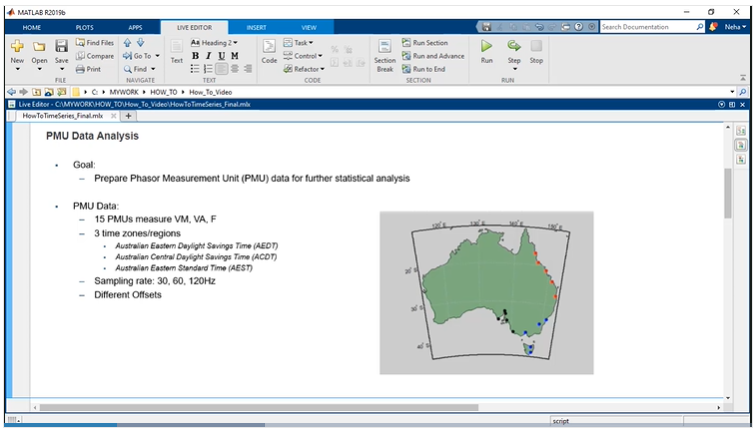# My training takes too long; what can I do to train my model faster?

You can do a couple of things within the Regression Learner app to speed up training. Just a quick reminder that I’m focusing on techniques within the app itself.

### Suggestion 1: Use parallel regression model training

The first and easier thing to try is to use parallel regression learner model training if you have the Parallel Computing ToolboxTM. This option creates a parallel pool to do parallel training and enables you to train multiple models simultaneously while continuing to work. If you select this option, you will see progress indicators on each training and queued model in the History list and you will also be able to cancel individual models at any time. This approach will help reduce the amount of time spent in training.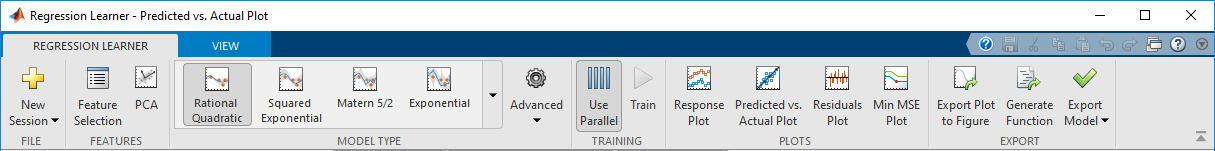Choose "Use Parallel" in the menu bar of the Regression Learner app.

### Suggestion 2: Use holdout validation

If your data is still very large, make sure you are using the right validation option. When you open a new session in the Regression Learner app and select the data, cross-validation is selected by default.

Cross-validation helps you partition your data into some number of folds (k), trains the model, and calculates the average test error over all folds. This method provides better protection against overfitting compared with other options, but requires multiple fits, so it works well for small and medium-sized data sets.

Holdout validation helps you select a percentage of the data to use as a testing set using the slider control. The app will train a model on the training set and assess its performance with the testing set. The model used for testing is based on only a portion of the data, so holdout validation is especially appropriate for large data sets.

You can of course also opt not to validate your model, but that opens you up to overfitting to the training data.
.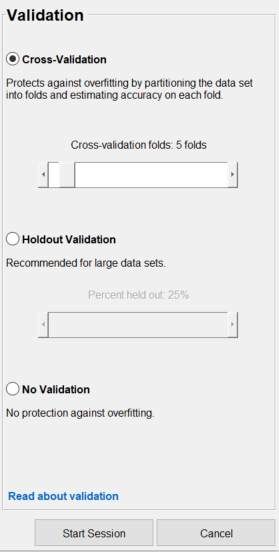Cross-validation is selected by default when you start a new session.

### Suggestion 3: Train your data on only one set of model types

If you know that your data will work well in one particular group model, or one particular group model is too slow to train, you can check or uncheck that particular type instead of training them all in the app. If you are not sure, you can try the option all quick-to-train, which will train all model types that are typically quick to train. You can then train all the models of the particular group that got the lowest root-mean-square error (RMSE) to find the best one.
.

### Suggestion 4: Slim down your training data

So often you hear about how to get enough data, but the key here is making sure you have enough of the right data. It might be possible that you have some unnecessary historic data from years ago that is not useful anymore. Removing or reducing this kind of data can speed up training, though of course you’ll want to keep an eye on accuracy and representation of data. This should be your last option since you have to be very cautious about reducing your data.

# How can I interpret the results of the different models and the plots available?

This is a very good question! There are a few steps to take after you finish training a model with your data to interpret results.

Step 1: Find the model with the smallest root-mean-square error

RMSE measures the distance between the predicted values and the values observed for each model, so it measures how spread these residuals are. The app will add a box around the lowest RMSE.

Step 2: Explore the model

Once you select the model with the lowest RMSE, the next step is to check the
different plots available
in the app. Two popular plots are response and predicted vs. actual.

In the image: The lower RMSE will be in bold and have a box around it.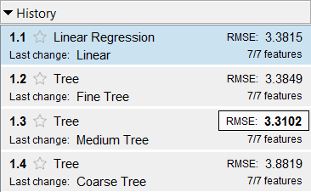The lower RMSE will be in bold and have a box around it.

#### Response plot

A response plot displays the predicted response against the observation in vertical lines. This plot is particularly interesting if you are using holdout validation or cross-validation for your data since the predictions displayed in the plot are for the held-out observations, which the model has not been trained with.

In the image: See the distance between predictions and observations using a response plot.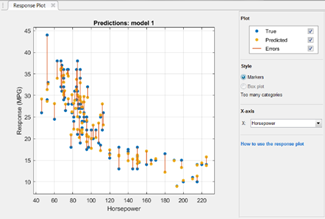See the distance between predictions and observations using a response plot.

#### Predicted vs. actual plot

A predicted vs. actual plot helps you check the model performance. The predicted response of your model is plotted against the actual, true response. In this plot, a perfect regression model would have a predicted response the same as the observed values, so all the points would lie on the diagonal line. However, this doesn’t happen in real life, so the goal is for the points to be as close to the diagonal line as possible and scattered roughly symmetrically around the line. If you detect patterns in the plot, that means that the model can be improved, and you can either train another model type or make the model more flexible using advanced options.

In the image: Predicted vs actual plot helps you visualize the accuracy of a regression model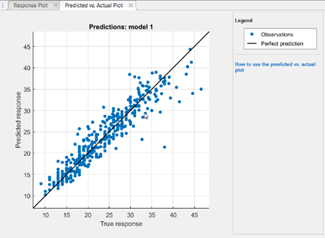Predicted vs. actual plot helps you visualize the accuracy of a regression model.

## I chose the model with the lowest RMSE; is there anything else I should do to optimize it?

After you have trained and assessed an initial model for your data, you can ensure the best performance by tuning the hyperparameters of your model.

To automatically select and find the best hyperparameter values for the model, you can use the
hyperparameter optimization feature
. The app tries different combinations of hyperparameter values to minimize the model’s mean squared error (MSE) and returns a new model with the optimized hyperparameters.

Since the impact of hyperparameter tuning varies across models, you have to optimize the hyperparameters for multiple types of models because your initial model may not yield optimal performance.

## How can I start making predictions?

To make predictions on new data using your fully trained and optimized model, you’ll need to send the model somewhere. You can export it to the workspace in MATLAB, or generate MATLAB code for training a model using the same steps you did in the app. From there, you can deploy the model using MATLAB Compiler™ or generate C/C++ code from the model using MATLAB Coder™.

See an example to find out what steps to follow
.